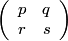#### Factorization of a 2x2 non-singular non-negative matrix in the style of Perron, Band 1

Input: a matrix A =, p, q, r, s ≥ 0 with p ≥ q or r ≥ s and Δ= ps – qr ≠ 0.
We express A uniquely as BC, where
1. B=[bij] is unimodular and b11 ≥ b12 and b21 ≥ b22,
2. C=[cij] is upper triangular with (a) c11 > 0, c22 > 0 and (b) -c22 ≤ c12 ≤ c11.
This factorization was Lemma 3 in K.R. Matthews and R.F.C. Walters, Some properties of the continued fraction expansion of (m/n)e 1/q, Proc. Camb. Phil. Soc. 67 (1970) 67-74 and is a version of Satz 4.1. of Die Lehre von den Kettenbrüchen, Band 1, S. 111.
(Lemma 3 of that paper stated that -c22 < c12 ≤ c11, which is true if q > 0 or s > 0.)

Here c11 = gcd(p, r), b11 = p/gcd(p, r), b21 = r/gcd(p, r), c11c22=|Δ|.
If b21 > 1 and p/r = [a0, ..., an] = An/Bn, an > 1, then b12/b22 = An - 1/Bn - 1 or (An – An - 1)/(Bn – Bn - 1).
If b21 = 1, then b22 = 0 and b12 = 1 if Δ < 0, whereas b22 = 1 and b12 = b11 – 1 if Δ > 0.
Finally, c12 is determined by q = b11c12 + b12c22.

Enter p (≥ 0):
Enter q (≥ 0):
Enter r (≥ 0):
Enter s (≥ 0):